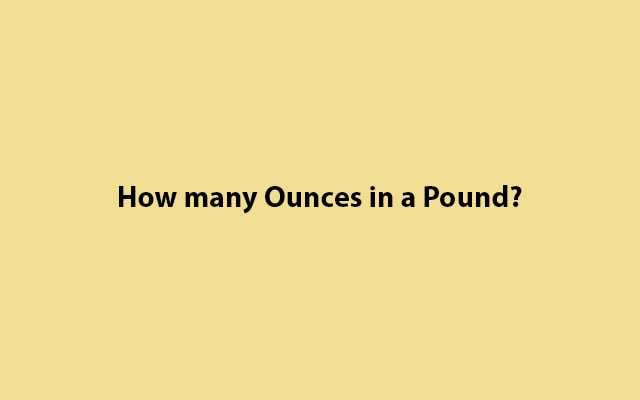# How many Ounces in a Pound? (oz in lb) With Example

Do you have a question about How many Ounces in a Pound (Oz in Lb)? You can find your answer below with simple examples. Also, you can find Pounds to Ounces conversion table.Firstly, let’s understand the values and relation between Ounces and Pounds.

Secondly, we will take some examples to know more in deep.

Relation: 1 pound (lb) is equal to 16 ounces (oz).

### 1 lb = 16 oz

So, we have to multiply by 16 to count pounds to ounces.

#### How to convert?

Use this formula to convert. Here “m” defines the mass.

m(oz) = m(lb) × 16

## How many Ounces in a Pound?

You can understand deeply it with the following examples.

Example #1 (Convert 8 lb to ounces:)

• m(oz) = 8 x 16 = 128(oz)

Example #2 (Convert 12 lb to ounces:)

• m(oz) = 12 x 16 = 192(oz)
##### Pounds to Ounces conversion table
Pounds (lb) Ounces (oz)
0 lb 0 oz
0.1 lb 1.6 oz
1 lb 16 oz
2 lb 32 oz
3 lb 48 oz
4 lb 64 oz
5 lb 80 oz
6 lb 96 oz
7 lb 112 oz
8 lb 128 oz
9 lb 144 oz
10 lb 160 oz
20 lb 320 oz
21 lb 336 oz
22 lb 352 oz
23 lb 368 oz
24 lb 384 oz
25 lb 400 oz
26 lb 416 oz
27 lb 432 oz
28 lb 448 oz
29 lb 464 oz
30 lb 480 oz
40 lb 640 oz
50 lb 800 oz
60 lb 960 oz
70 lb 1120 oz
80 lb 1280 oz
90 lb 1440 oz
100 lb 1600 oz
1000 lb 16000 oz

Above all, are the conversation results between 0 to 1000 (lb) with ounces values. Even more, you can convert ounces to pounds by vice versa. Just divide ounces by 16 or multiply by 0.0625.

Related